### Fundamentals of Signal Processing

Data is displayed for academic year: 2023./2024.

#### Course Description

In this course students gain fundamental signal processing knowledge with the aim of understanding the methods and the algorithms of digital signal processing.
The following topics are covered: Signals. Classification of signals. Signal decomposition. Fourier transforms. Signal spectrum. Sampling and reconstruction. The sampling theorem. Window functions and spectral analysis. Discrete cosine transform. Systems. Classification of systems. Linear time invariant systems. The convolution sum. Laplace and Z transforms. Transfer function and frequency response. Equivalence of continuous and discrete systems. Euler and reversed Euler methods. Bilinear transform. Filtering, prediction and reconstruction of signals. Amplitude-selective filters. Filter design problem. Phase and group delay. Linear-phase systems. All-pass systems. Classification of digital filters. Computer aided design of amplitude-selective filters. Fast Fourier transform and its applications. Linear and circular convolution. Efficient computation of the convolution sum. Digital signal processor. Fixed-point arithmetic. Signal quantization.

#### Study Programmes

[FER3-EN] Computing - study
Elective Courses (5. semester)
[FER3-EN] Electrical Engineering and Information Technology - study
Elective Courses (5. semester)
[FER3-EN] Data Science - profile
(1. semester)

#### Learning Outcomes

1. Classify signaly and systems by type
2. Explain the importance of signal processing in computing, electronics, control engineering and telecommunications
3. State and explain the Nyquist-Shannon sampling theorem
4. Analyze signals using their spectrum
5. Analyze systems using theirs transfer function and frequency response
6. Explain the equivalence between time continuous and time discrete systems
7. Explain signal filtration
8. Design a basic digital filter using a computer
9. Explain what the fast Fourier transform is and list its applications

#### Forms of Teaching

Lectures

Lectures present theoretical concepts.

Exercises

Recitations include solving practical tasks and discussing solving procedures.

Laboratory

Laboratory exercises introduce to students how computers are used for signal processing.

Continuous Assessment Exam
Laboratory Exercises 50 % 15 % 50 % 15 %
Homeworks 0 % 5 % 0 % 0 %
Mid Term Exam: Written 0 % 30 % 0 %
Final Exam: Written 0 % 30 %
Final Exam: Oral 20 %
Exam: Written 50 % 50 %
Exam: Oral 35 %
##### Comment:

Mandatory prerequisites for oral exams are achieving at least 50% of points on midterm and final exam combined, or on the written part of a regular exam, and at least 50% on laboratory exercises.
A minimum of 4 points is required to pass the final oral exam.
A minimum of 8 points is required to pass the regular oral exam.

#### Week by Week Schedule

1. Introduction. Signals. Classification of signals. Signal decomposition.
2. Fourier transforms (DFT, DTFT, CTFT). Signal spectrum. Sampling and reconstruction. The sampling theorem.
3. Window functions and spectral analysis. Discrete cosine transform (DCT-II).
4. Systems. Classification of systems. Linear time invariant systems. The convolution sum.
5. Laplace transform. Z transform. Transfer function and frequency response.
6. Equivalence of continuous and discrete systems. Euler and reversed Euler methods. Bilinear transform.
7. Signal filtering, reconstruction and prediction. Amplitude-selective filters.
8. Midterm exam
9. Digital filters. Filter specification. Phase and group delay.
10. Classification of digital filters. Linear-phase systems. All-pass systems.
11. Computer aided design of amplitude-selective linear-phase FIR filters.
12. Computer aided design of amplitude-selective minimum-phase IIR filters.
13. Fast Fourier transform and its applications. Linear and circular convolution. Efficient computation of the convolution sum.
14. Filter realizations. Fixed-point arithmetic. Digital signal processors.
15. Final exam

#### Literature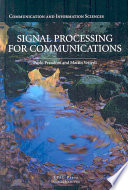Paolo Prandoni, Martin Vetterli (2008.), Signal Processing for Communications, EPFL Press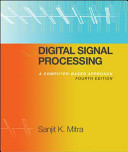Sanjit Kumar Mitra (2010.), Digital Signal Processing: A Computer Based Approach, McGraw-HillAlan V. Oppenheim, Ronald W. Schafer (2010.), Discrete-Time Signal Processing, Pearson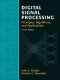John G. Proakis, Dimitris G. Manolakis (2007.), Digital Signal Processing, Pearson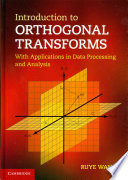Ruye Wang (2012.), Introduction to Orthogonal Transforms, Cambridge University Press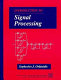Sophocles J. Orfanidis (1996.), Introduction to Signal Processing, Prentice Hall

#### General

ID 223374
Winter semester
5 ECTS
L2 English Level
L1 e-Learning
45 Lectures
0 Seminar
10 Exercises
20 Laboratory exercises
0 Project laboratory